# Rajasthan Board Class 12 Maths Important Questions And Answers

Class 12 is a very crucial year for the students of Rajasthan Board as it is the time for them to acquire more in-depth knowledge in their stream of choice. A student who has passed the year successfully can then go for further studies or professional colleges of their choice. In order to score well, Science stream students will then have to practice hard and learn the concepts of subjects like Maths, Physics, Chemistry and Biology thoroughly. Rajasthan Board Class 12 Maths Important Questions is a significant resource for the students.

To help the students with their preparation for the exams of Rajasthan Board Class 12 , we have compiled here some important questions from the Class 12 Maths. These questions will help the students to also self- assess their current preparation level. Students can solve these RBSE Class 12 Important Questions of Mathematics and gain the confidence to face the exams fearlessly.

We have prepared these after careful analysis of the previous year question papers and sample papers so that students get an idea about the type of questions asked in Board exams.

### Rajasthan Board Class 12 Maths Important Questions And Answers

Question 1: If the points (x, -2), (5, 2), (8, 8) are collinear, then find the value of x.

Solution:

If (x, -2), (5, 2), (8, 8) are collinear, then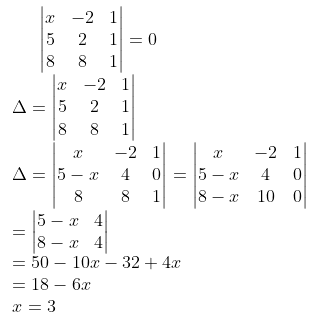Question 2: Find the unit vector along with the sum of vectors a = 2i + 2j – 5k and b = 2i + j +3k.

Solution:

The sum of the given vectors is vector (a + b) ( = vector c, say) = 4i + 3j – 2k and |vector c| = √(42 + 32 + (-2)2) = √29

Thus, the required unit vector is

c = [1 / |c|] [c] = (1 / √29) [4i + 3j – 2k]

= [4 / √29] i + [3 / √29] j – [2 / √29]k

Question 3: If 2 P (A) = P (B) = 5 / 13 and P (A / B) = ⅖ then find P (A ∪ B).

Solution:

2 P (A) = P (B) = 5 / 13

P (A) = (1 / 2) * (5 / 13) = 5 / 26

P (B) = 5 / 13

P (A / B) = ⅖

P (A / B) = P (A ⋂ B) / P (B)

(2 / 5) = P (A ⋂ B) / (5 / 13)

P (A ⋂ B) = (2 / 5) * (5 / 13)

P (A ⋂ B) = 2 / 13

P (A ∪ B) = P (A) + P (B) – P (A ⋂ B)

= (5 / 26) + (5 / 13) – (2 / 13)

= (5 / 26) + (6 / 26)

= 11 / 26

Question 4: Find A, if 2A –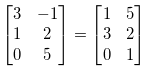.

Solution: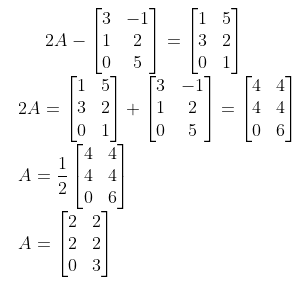Question 5: Find the direction cosines of the line (x – 2) / 2 = (y + 1) / -2 = (z – 1) / 1.

Solution:

The line is (x – 2) / 2 = (y + 1) / -2 = (z – 1) / 1 — (i)

(a, b, c) = (2, -2, 1)

The direction ratios are ± a / √a2 + b2 + c2, ± b / √a2 + b2 + c2, ± c / √a2 + b2 + c2

= ± 2 / √4 + 4 + 1, ± (-2) / √4 + 4 + 1, ± 1 / √4 + 4 + 1

= ± 2 / 3, ± 2 / 3, ± 1 / 3

Question 6: Find ∫[tanx / cot x] dx.

Solution:

∫[tan x / cot x] dx

tan x = 1 / cot x

= ∫tan2 x dx

= ∫sec2 x dx – ∫1 dx

= tan x – x + c

Question 7: Find the angle between planes r . (i – j + k) = 5 and r . (2i + j – k) = 7.

Solution:

Let the normal of the planes be a = i – j + k and b = 2i + j – k.

a . b = |a| . |b| cos θ

cos θ = (a . b) / |a| . |b|

cos θ = [i – j + k] [2i + j – k] / |i – j + k| |2i + j – k|

= [2 – 1 – 1] / √1 + 1 + 1 √4 + 1 + 1

cos θ = 0

θ = cos-1 0

θ = 𝛑 / 2

Question 8: From a pack of 52 cards, two cards are drawn randomly one by one without replacement. Find the probability that both of them are red.

Solution:

The probability of getting the first card as red is 26 / 52 as there are 26 red cards in a deck.

Now the probability of getting the second card as red is 25 / 51, as 25 red cards are left and the total number of cards is 51.

P (both the cards are red) = [26 × 25] / [52 × 51]

= 650 / 2652

= 25 / 102

Question 9: Show the region of a feasible solution under the following constraints 2x + y ≥ 8, x ≥ 0, y ≥ 0 in the answer book.

Solution: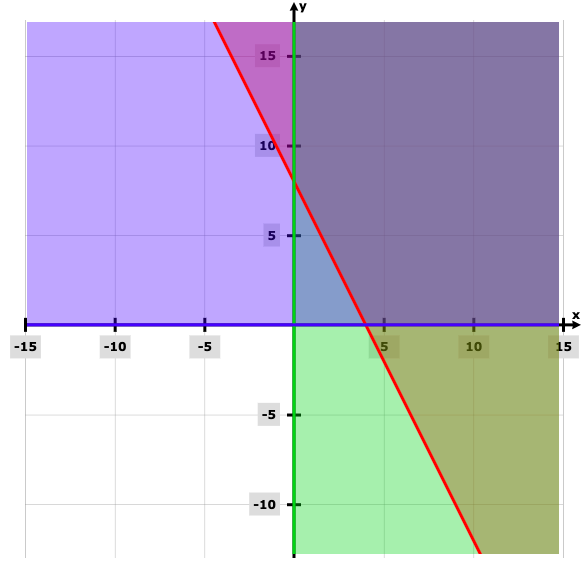Question 10: Examine the continuity of function f (x) =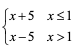at point x = 1.

Solution:

f (x) =Left hand limit = lim x→1- (x + 5) = 6

Right hand limit = lim x→1- (x – 5) = – 4

Since LHL ≠ RHL, f (x) is discontinuous at x = 1.

Question 11: Find ∫(1 / 1 + sinx) dx.

Solution:

∫(1 / 1 + sinx) dx

= ∫(1 – sinx) / (1 + sinx) (1 – sinx) dx

= ∫(1 – sinx) / (12 – sin2 x) dx

= ∫(1 – sinx) / cos2 x dx

= ∫(1 / cos2 x) dx – (sinx / cos2 x) dx

= ∫[sec2 x – secx tanx] dx

= tanx – secx + c

Question 12: If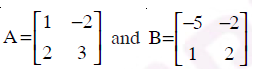, then find 2A2 – 3B.

Solution: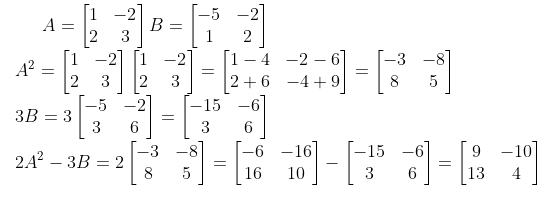Question 13: Examine the continuity of function f defined by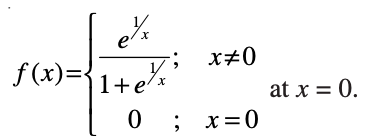Solution:

f (0) = 0

f (0 – 0) = lim h→0 f (0 – h)

= lim h→0 e([1 / 0] – h) / [1 + e([1 / 0] – h)]

= lim h→0 e-1 / h / [1 + e-1 / h]

= e-∞ / [1 + e-∞]

e-∞ = 1 / [e] = 1 / ∞ = 1 / (1 / 0) = 0 / 1 = 0

f (0 – 0) = 0 / [1 + 0] = 0 / 1 = 0

f (0 + 0) = lim h→0 f (0 + h)

= lim h→0 e1 / h / [1 + e1 / h)]

= lim h→0 e1 / h / e1 / h [e-1 / h + 1]

= lim h→0 1 / [e-1 / h + 1]

= 1 / [1 + e-∞]

= 1 / 0 + 1

= 1

f (0) = f (0 – 0) ≠ f (0 + 0)

So, f (x) is not continuous at x = 0.

Question 14: Prove that the relation R in a set of real numbers R defined as R = {(a, b): a ≥ b} is reflexive and transitive but not symmetric.

Solution:

R = {(a, b) : a ≥ b}

Reflexive

R = {(a, a) : a > a}

Hence, R is reflexive.

Symmetric

Let (a, b) ∈ R

aRb ⇔ a ≥ b

bRa ⇔ b ≥ a not true

(b, a) ∉ R

Hence, R is not symmetric.

Transitive

Let (a, b) ∈ R and (b, c) ∈ R

aRb ⇔ a ≥ b

bRc ⇔ b ≥ c

a ≥ c

(a, c) ∈ R

Hence, R is transitive.

So, R is reflexive and transitive but not symmetric.

Question 15: Solve 2 tan-1 (sin x) = tan-1 (2sec x), 0 < x < π / 2.

Solution:

2 tan-1 (sin x) = tan-1 (2 sec x)

2 tan-1 x = tan-1 (2x / (1 – x))

tan-1 [(2 sin x) / (1 – sin2 x)] = tan-1 [2 sec x]

(2 sin x) / (1 – sin2 x) = 2 sec x

sin x / cos2 x = sec x

sin x sec x = 1

sin x / cos x = 1

tan x = 1

x = 𝛑 / 4

Question 16: Find the intervals in which the function f given by f (x) = x2 – 6x + 5 is

a) Strictly increasing

b) Strictly decreasing

Solution:

f (x) = x2 – 6x + 5

f ‘ (x) = 2x – 6

Put f ‘ (x) = 0

2x – 6 = 0

x = 3

Then divide the given interval into two parts as (-∞, 3) and (3, ∞).

[i] Take the interval (3, ∞)

f ‘ (x) = 2x – 6 > 0

The given function is strictly increasing in the interval (3, ∞).

[ii] Take the interval (-∞, 3)

f ‘ (x) = 2x – 6 < 0

The given function is strictly decreasing in the interval (-∞, 3).

Question 17: Prove that the relation R defined on set Z as a R b ⇔ a − b is divisible by 3, is an equivalence relation.

Solution:

aRb ⇔ a – b is divisible by 3

(i) Reflexive : aRa ⇒ a – a = 0 is divisible by 3

∴ R is reflexive.

(ii) Symmetric: Let (a, b) ∈ R

⇒ aRb ⇔ a – b is divisible by 3

⇒ bRa ⇔ b – a is divisible by 3

⇒ (b, a) ∈ R

∴ R is symmetric

(iii) Transitive: Again (a, b) ∈ R and (b, c) ∈ R

aRb ⇔ a – b is divisible by 3 and bRc ⇔ b – c is divisible by 3

⇒ a – b is divisible by 3 and b – c is divisible by 3

⇒ (a – b) + (b – c) is divisible by 3

⇒ a – c is divisible by 3

⇒ (a, c) ∈ R

∴ R is transitive, hence R is an equivalence relation.

Question 18: Prove that cos-1 (63 / 65) + 2 tan-1 (1 / 5) = sin-1 (3 / 5).

Solution:

LHS = cos-1 (63 / 65) + 2 tan-1 (1 / 5)

Take θ = cos-1 (63 / 65)

cos θ = 63 / 65

sin θ = √1 – cos2 θ

= √1 – (63 / 65)2

sin θ = 16 / 65

θ = sin-1 (16 / 65)

= sin-1 (16 / 65) + tan-1 (2 * (1 / 5)) / (1 – (1 / 25))

= sin-1 (16 / 65) + tan-1 (10 / (25 – 1))

= sin-1 (16 / 65) + tan-1 (5 / 12)

θ = tan-1 (5 / 12)

tan θ = 5 / 12

sec2 θ = 1 + tan2 θ

= 1 + (25 / 144)

= 169 / 144

sec θ = 13 / 12

cos θ = 12 / 13

sin θ = √1 – (12 / 13)2

= √1 – [144 / 169]

= √25 / 169

= 5 / 13

= sin-1 (16 / 65) + sin-1 (5 / 13)

sin-1 x + sin-1 y = sin-1 [x + √1 – y2 + y √1 – x2]

= sin-1 [(16 / 65) (√1 – (5 / 13)2) + (5 / 13) (√1 – (16 / 65)2)

= sin-1 ((16 / 65) * (12 / 13) + (5 / 13) * (63 / 65))

= sin-1 ([192 + 315] / (63 / 65))

= sin-1 [507 / (65 * 13)]

= sin-1 (3 / 5)

Question 19: If the radius of a sphere is measured as 7 cm with an error of 0.01 cm, then find the approximate error in calculating its volume.

Solution:

r = 7cm

Δr = 0.01 cm

V = (4 / 3) 𝛑r3

dv / dr = (4 / 3) 𝛑3r2

= 4𝛑r2

= 4𝛑 * 49

= 196 𝛑 cm3 / cm

Δv = [dv / dr] * (Δr)

Δv = [196 𝛑] * (0.01)

= 1.96 𝛑

Question 20: Find two positive numbers x and y, the sum of them is 60 and xy3 is maximum.

Solution:

Let P = xy3

It is given that x + y = 60

⇒ x = 60 − y

P = (60 − y) y3 [Putting value of x]

= 60y3 − y4

⇒ dP / dy = 180y2 − 4y3

d2P / dy2 = 360y − 12y2

For maximum or minimum values of y, P we have

dy / dP = 0

⇒ 180 y2 − 4y3 = 0

⇒ 4y2 (45 − y) = 0

⇒ y = 0

45 − y = 0

y = 45

(d2P / dy2)y = 45 = 360 × 45 − 12(45)2

= 12 × 45 − (30 − 45)

= −8100 < 0

P is maximum when y = 45

When y = 45,

x + y =60

⇒ x = 60 − 45

x = 15

The numbers are 15 and 45.

Question 21: Solve the equation cos-1 x + cos-1 2x = (2π) / 3.

Solution:

cos-1 x + cos-1 2x = 2𝛑 / 3

cos-1 x + cos-1 y = cos-1 [xy – √1 – x2 √1 – y2]

cos-1 (x * 2x – √1 – x2 √1 – (2x)2) = 2𝛑 / 3

cos-1 (2x2 – √1 – x2 √1 – (2x)2) = 2𝛑 / 3

2x2 – √1 – x2 √1 – (2x)2 = 2𝛑 / 3

2x2 – √1 – x2 √1 – 4x2 = 2𝛑 / 3

2x2 + (1 / 2) = √1 – x2 √1 – 4x2

On squaring both sides,

(2x2 + (1 / 2))2 = (1 – x2) (1 – 4x2)

4x4 + 2x2 + (1 / 4) = 1 – 4x2 – x2 + 4x4

7x2 = 1 – (1 / 4)

7x2 = 3 / 4

x2 = 3 / 28

x = ± √3 / 28

The solution of cos-1 x + cos-1 2x = 2𝛑 / 3 is ± √3 / 28.

Question 22: Solve the following system of equations by using Cramer’s rule.

5x – 4y = 7

x + 3y = 9

Solution:

5x – 4y = 7 —- (1)

x + 3y = 9 —- (2)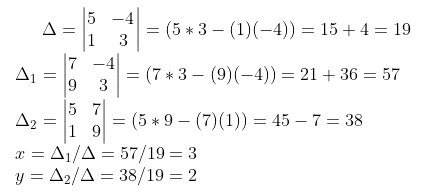Question 23: Using integration, find the area of a triangular region whose sides have the equations y = x + 1, y = 2x + 1 and x = 2. (Draw the figure in answer book)

Solution:

Consider y = x + 1 and y = 2x + 1

Equate both the equations and solve for x

x + 1 = 2x + 1

x = 0

Substitute x = 0 in y = x + 1,

y = 1

Substitute x = 2 in y = 2x + 1

y = 5

Graph of y = x + 1, y = 2x + 1 and x = 2 is given by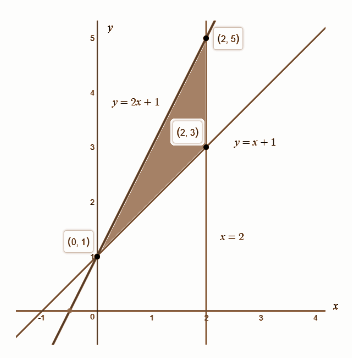Area = ∫02 [2x + 1 – x – 1] dx

= ∫02 x dx

= [x2 / 2]02

= 2 square units

Question 24: Find the area of the triangle whose vertices are A (1, 1, 1), B (1, 2, 3) and C (2, 3, 1).

Solution:

A (1, 1, 1), B (1, 2, 3) and C (2, 3, 1)

A (1, 1, 1), B (1, 2, 3)

AB = (1 – 1) i + (2 – 1) j + (3 – 1) k

= 0i + 1j + 2k

A (1, 1, 1), C (2, 3, 1)

AC = (2 – 1) i + (3 – 1) j + (1 – 1) k

= 1i + 2j + 0k

AB x AC = i [(1 x 0) – (2 x 2)] – j [(0 x 0) – (1 x 2)] + k [(0 x 2) – (1 x 1)]

= – 4i + 2j – 1k

|AB x AC| = √16 + 4 + 1 = √21

The area of the triangle ABC = (1 / 2) * (AB x AC)

= (1 / 2) * √21

= √21 / 2

Question 25: If x2 + y2 = t – (1 / t) and x4 + y4 = t2 + (1 / t2), then prove that x (d2y / dx2) + 2 (dy / dx) = 0.

Solution:

x2 + y2 = t – (1 / t)

x4 + y4 + 2x2y2 = t2 + (1 / t2) – 2t * (1 / t)

t2 + (1 / t2) + 2x2y2 = t2 + (1 / t2) – 2

x2y2 = – 1

2xy2 + 2x2y (dy / dx) = 0

xy (y + x (dy / dx) = 0

xy ≠ 0

y + x (dy / dx) = 0

(dy / dx) + (dy / dx) + x (d2y / dx2) = 0

x (d2y / dx2) + 2 (dy / dx) = 0

Question 26: A man is known to speak the truth 3 out of 4 times. He throws a die and reports that it is 6. Find the probability that it is actually 6.

Solution:

Let E1 be an event of a man telling the truth and E2 be an event of a man not telling the truth.

A = probability on 6 in the coin

P (E1) = 2 / 3

P (E2) = 1 / 3

P (A / E1) = 1 / 6

P (A / E2) = 5 / 6

P (E1 / A) = [(2 / 3) * (1 / 6)] / [(2 / 3) * (1 / 6) + (1 / 3) * (5 / 6)]

= 2 / 7

Question 27: Bag A contains 2 red and 3 black balls while another bag B contains 3 red and 4 black balls. One ball is drawn at random from one of the bags and it is found to be red. Find the probability that it was drawn from bag B.

Solution:

Consider the events E and F as follows:

E: Bag A is selected

F: Bag B is selected

A: Ball drawn is red

Since there are only two bags.

Bag A contains 2 red and 3 black balls

P (A / E) = 2 / 5

Bag B contains 3 red and 4 black balls

P (A / F) = 3 / 7

The required probability is

P (F / A) = [P (F) P (A / F)] / [P (E) P (A / E) + P (F) P (A / F)]

= (1 / 2) (3 / 7) / [(1 / 2) * (2 / 5) + (1 / 2) * (3 / 7)]

= (8 / 35) / [(3 / 14) + (8 / 35)]

= (3 / 14) / (29 / 70)

= 15 / 29

Question 28: Prove that if a plane has the intercepts a, b, c and is at distance p units from the origin, then prove that (1 / a2) + (1 / b2) + (1 / c2) = (1 / p2).

Solution:

The plane is (x / a) + (y / b) + (z / c) = 1

Perpendicular distance from origin (0, 0, 0)

P = |0 + 0 + 0 – 1| / √(1 / a2 + 1 / b2 + 1 / c2)

= 1 / √(1 / a2 + 1 / b2 + 1 / c2)

(1 / a2 + 1 / b2 + 1 / c2) = 1 / p2

Question 29: A dice is thrown twice and the sum of the numbers appearing is observed to be 7. Find the conditional probability that the number 2 has appeared at least once.

Solution:

A die is thrown twice and the sum of numbers appearing is observed to be 7.

When a dice is rolled twice, the outcome is as follows:

(1, 1) (1, 2) (1, 3) (1, 4) (1, 5) (1, 6)

(2, 1) (2, 2) (2, 3) (2, 4) (2, 5) (2, 6)

(3, 1) (3, 2) (3, 3) (3, 4) (3, 5) (3, 6)

(4, 1) (4, 2) (4, 3) (4, 4) (4, 5) (4, 6)

(5, 1) (5, 2) (5, 3) (5, 4) (5, 5) (5, 6)

(6, 1) (6, 2) (6, 3) (6, 4) (6, 5) (6, 6)

Probability = Favourable outcome / Total number of outcomes

Favorable outcome that number 2 has appeared at least once :

(2, 1) (2, 2) (2, 3) (2, 4) (2, 5) (2, 6) (1, 2) (3, 2) (4, 2) (5, 2) (6, 2)

Favorable outcomes = 11

Total number of outcome = 36

P (number 2 has appeared at least once) = 11 / 36

Question 30: Prove that the lines r = i + j – k + λ (3i – j) and r = 4i – k + μ (2i + 3k) are intersecting, also find the point of intersection.

Solution:

The position vectors of arbitrary points on the given lines are

(i + j – k) + λ (3i – j) = (3λ + 1)i + (1 – λ) j – k

If the lines intersect, then they have a common point. So, for some values of λ and μ,

(3λ + 1) i + (1 – λ) j – k = (2μ + 4)i + 0j + (3μ – 1)k

(3λ + 1) = (2μ + 4); 1 – λ = 0; -1 = (3μ – 1)

Solving the last two of these three equations, λ = 1 and μ = 0.

These values of λ and m satisfy the first equation.

So, the given lines intersect.

Putting λ = 1 in first line,

r = (i + j – k) + (3i – j) = 4i + 0j – k which is the position vector of the point of intersection.

Thus, the coordinates of the point of intersection are (4, 0, −1).